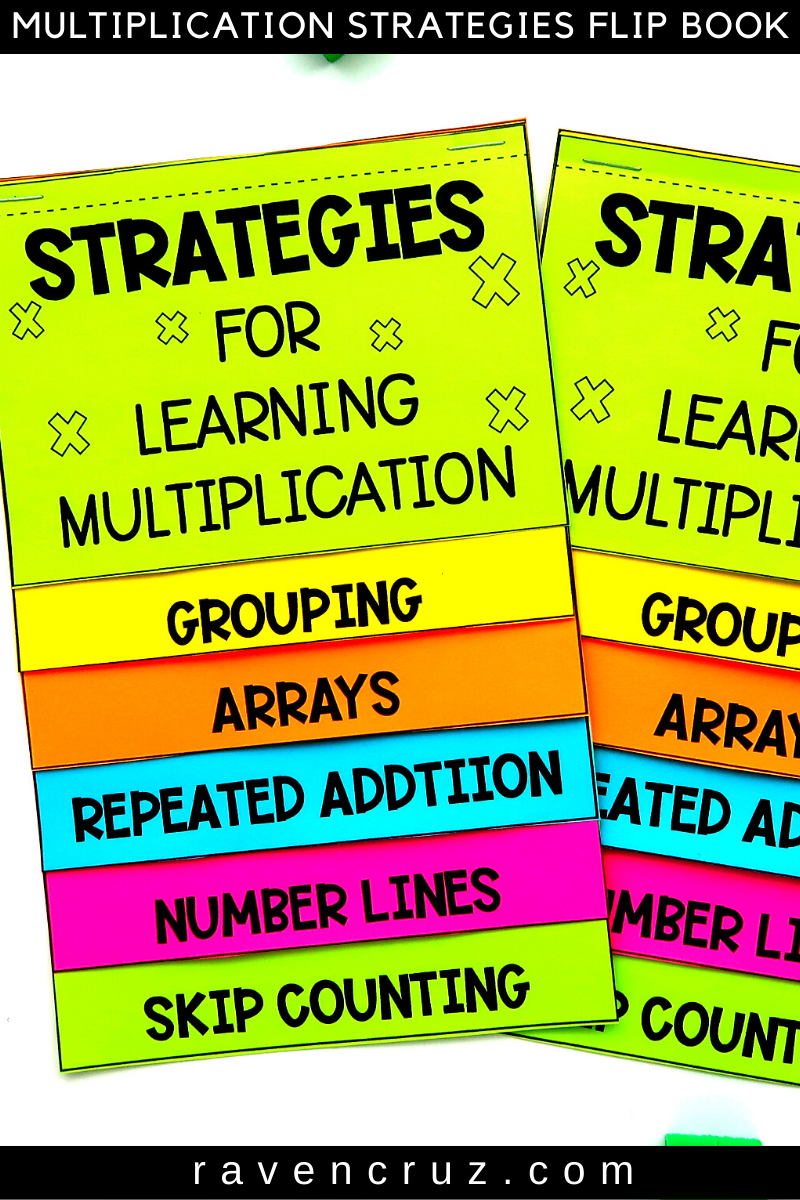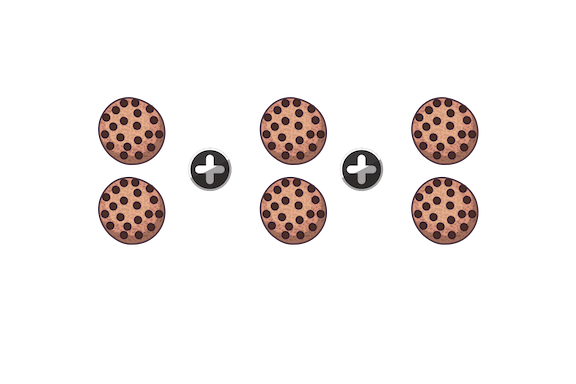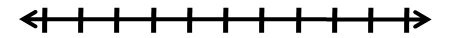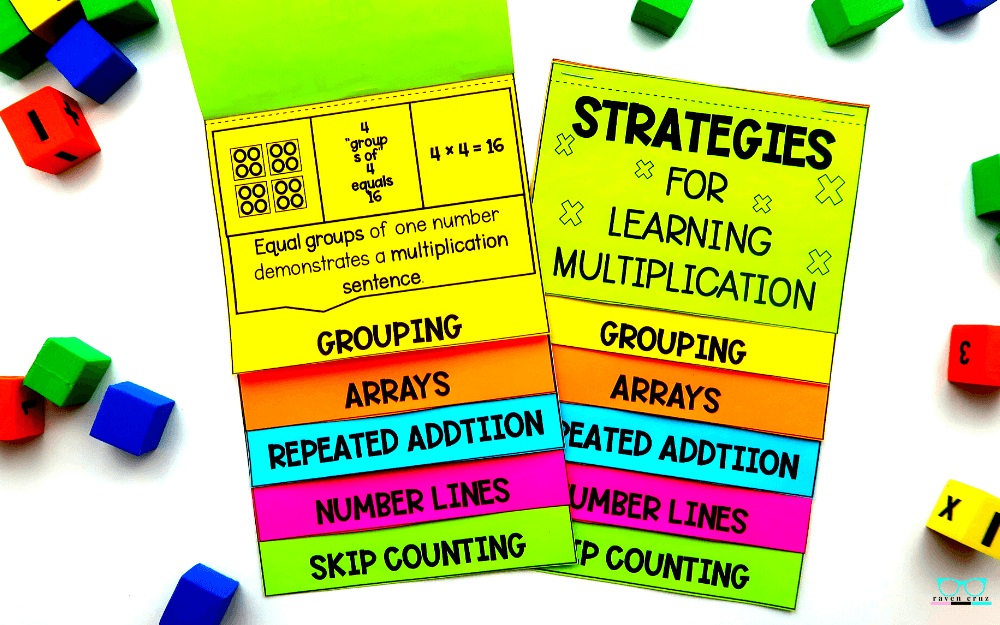One Epic Multiplication Strategies Flip Book for 3rd Grade - Raven Cruz

# One Epic Multiplication Strategies Flip Book for 3rd Grade

I love flip books! There is just something extremely satisfying about putting together a beautiful + functional manipulative for the classroom. Honestly, that is why I call my favorite flip book the one epic multiplication strategies flip book for 3rd grade. Yes, I'm serious!

Let me tell MORE about WHY:

The multiplication strategies flip book covers: equal groups, arrays, repeated addition, number lines, skip counting.

Each strategy has 3 examples and a definition. For example, equal groups includes a model of 4 equal groups of 4, explains that "4 groups of 4 equals 16", and a number sentence that explains 4 x 4 = 16​.

Consequently, this flip book is the #1 BEST SELLER in my Teachers Pay Teachers store!## How to use Multiplication Strategies Flip Book

There are many situations that this flip book can be beneficial in your classroom. Next, I will list few ideas, and if you have a brilliant idea that you want me to share, you can leave them in the comments below or tag me on Instagram @mrs.raven.cruz or Facebook Math with Raven with a photograph of how you used them in your class/homeschool. You never know, I may just share your photo.

• Interactive notebooks
• Math centers
• Rotations
• Small Groups
• Math Tool Boxes
• For Intervention

## Multiplication by Grouping

The first multiplication strategy is learning multiplication by grouping is the process of separating objects into equal groups. Most commonly when using equal groups, repeated addition is also used. This method is one of the most straightforward ways for students to comprehend the strategy of multiplication by grouping.

As you can see in the example below there are 3 equal groups of 2 cookies. Students can use repeated addition to solve a multiplication problem like the one below.

In the classroom teachers can use manipulative such as; erasers, counters, small blocks, or even drawing on dry erase boards to practice the strategy of grouping and repeated addition.2 + 2 + 2 = 6

## Multiplication by Arrays

Second, are arrays. Arrays are an arrangement of objects arranged in rows and columns.

The arrangement of cookies shown below shows the systematic way to introduce arrays.  The student will first learn a 1 × 4 array, 2 × 4 array, 3 × 4 array, 4 × 4 array, and so on... A larger arrangement of objects can also be broken down into arrays to preform many different types of multiplication, such as properties of multiplication.

Also, making arrays in the classroom can be fun AND engaging for students. Using colorful objects, such as; erasers, counters, and blocks to build arrays can keep all types of learners engaged and ready to learn.1 × 42 × 43 × 44 × 4

Third, is repeated addition. We touched on using repeated addition with grouping above when we grouped 2 cookies in groups of three. Both learning using grouping and learning using arrays are complimentary to each other.

3 + 3 + 3 + 3 + 3 + 3 = 18

## Multiplication by Skip Counting

Last, learning multiplication by number lines uses the next strategy - skip counting. Skip counting is not just for providing students a way to count faster, it also helps them solve multiplication problems.

Starting at 0 students count in intervals until reaching the desired number. Students can also point out the pattern being used when skip counting.

0, 5, 10, 15, 20, 25, 30...

## Multiplication by Number Lines

Fourth, is learning multiplication by number lines. Students will take "jumps" on the number line. These jumps help students solve  multiplication problems by skip counting.

Number lines are an exceptional way to teach number sense as well.2  4  6  8 10.....

If you enjoy getting FREE quality elementary math resources + tips in your email-box, sign up for my monthly freebies and newsletter below. The freebies are EXCLUSIVE to my email subscribers.

### You may also like this blog post about 5 Strategies to Teach Multiplication with Ease.